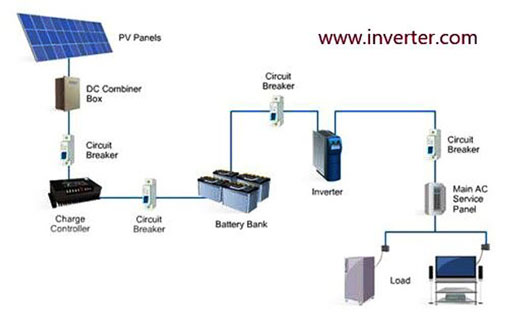# How to Size Batteries and Charge Controller in Off Grid Solar System?

As in off grid solar system, there is no access to the grid supply, there will be a mandatory need for batteries to supply our loads in cloudy days and at the night hours.

Let us see how can we size the batteries bank and charge controller:

First step:

We need to calculate how much energy our loads will need per day, and this can be done simply by knowing the wattage of each device and how many hours it works per day, multiply the wattage per each device by its run time and add all the wattage hours for all device, so can get total energy required for your home per day. Due to losses & inefficiencies, we recommend to multiply total watt hour by 1.5 factor.Second step:

We need to indicate batteries days of autonomy which means number of days your batteries bank will be able to handle your loads, and generally its 3-5 days.

Third step:

We shall multiply total watt hours per day getting from step one referred above by days of autonomy getting from second step referred above by 2 (Number 2 refers it should be 50% depth of charge in our batteries bank system, as it does not have to be fully discharged). The result of these 3 factors multiplication is the total watt hours required from the batteries bank. Divide this value by batteries voltage, we shall get batteries bank AH (ampere hour).

Fourth step:

We shall indicate how many batteries we shall use and how can we wire them up (parallel & series). In series batteries connection, the voltage will add up and in parallel connections the amperage will add up, so you can make such configuration as you like but keep in mind to achieve total AH calculated in third step and batteries bank voltage. Generally indicating the number of batteries is depending on how you will configure, we mean how many batteries will be in series and how many batteries will be in parallel.

Also, keep in mind to use brand new batteries for all, as if you use only one used old battery, then it will degrade and reduce the life span for the whole batteries system.

Fifth step:

Here we shall indicate charge controller current and voltage. By dividing total solar array power "watt" on batteries voltage and multiply the result by 1.25 oversizing (safety) factor, we shall get charge controller output rated current.